#Function Repository Resource:

# FindExtraordinaryLines

Return the lines of three or more points, given a set of points

Contributed by: Ed Pegg Jr
 ResourceFunction["FindExtraordinaryLines"][pts] returns indices for sets of three or more points on the same line, given pts.

## Details and Options

For a finite set of points, a line going through only two points is called an ordinary line.

## Examples

### Basic Examples

Here are 11 points that make 15 lines of three points each:

 In:=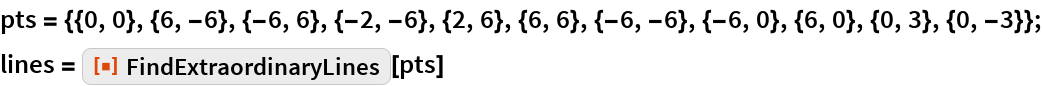Out=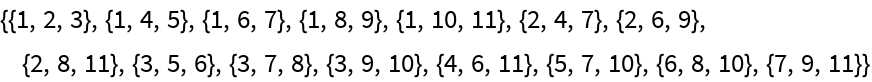A graphic showing the points and lines:

 In:=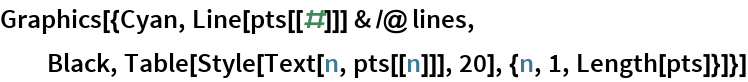Out=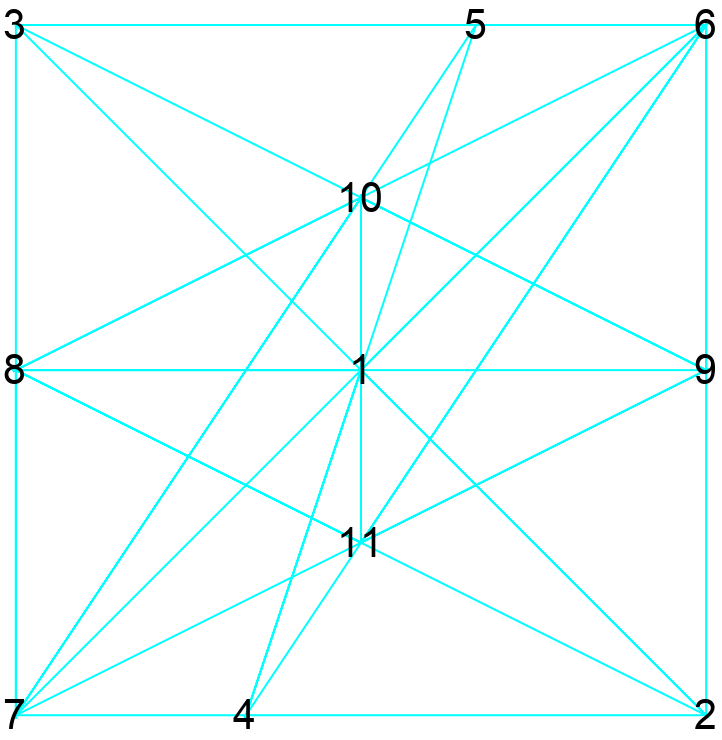### Scope

Here is a set of 20 lattice points where all lines are ordinary:

 In:=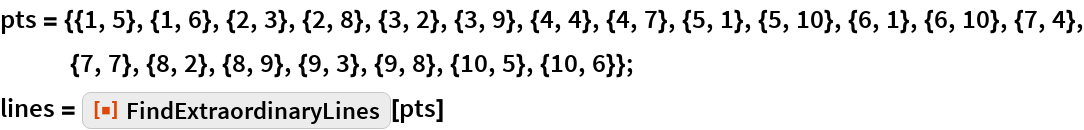Out=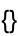In:=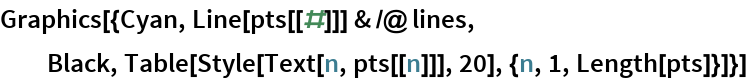Out=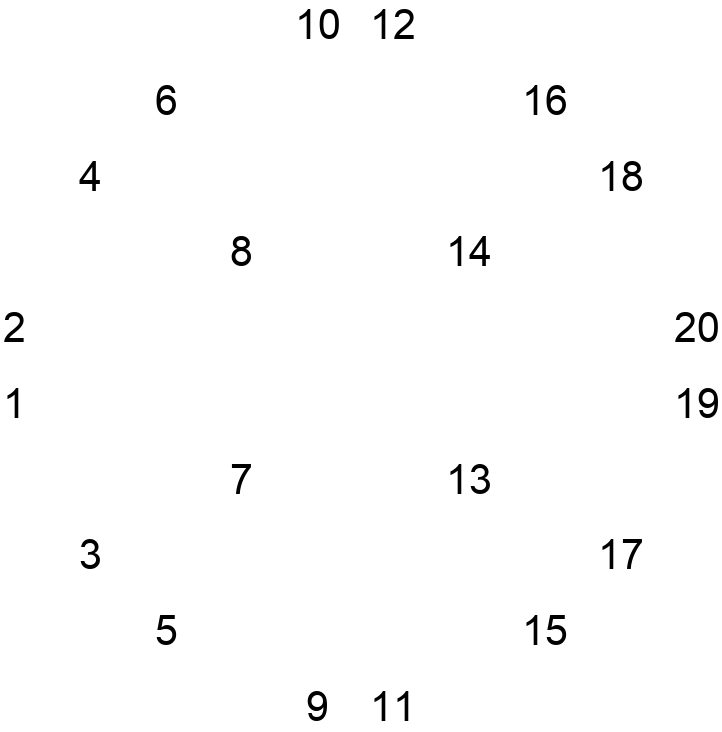Most sets of 20 points from this lattice will have many extraordinary lines:

 In:=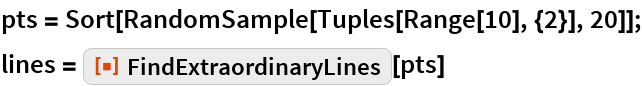Out=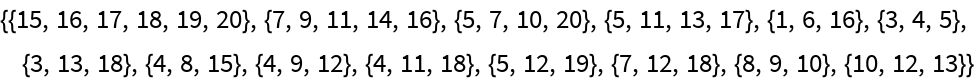In:=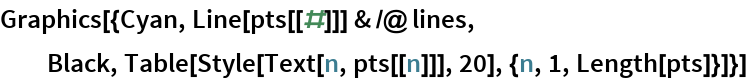Out=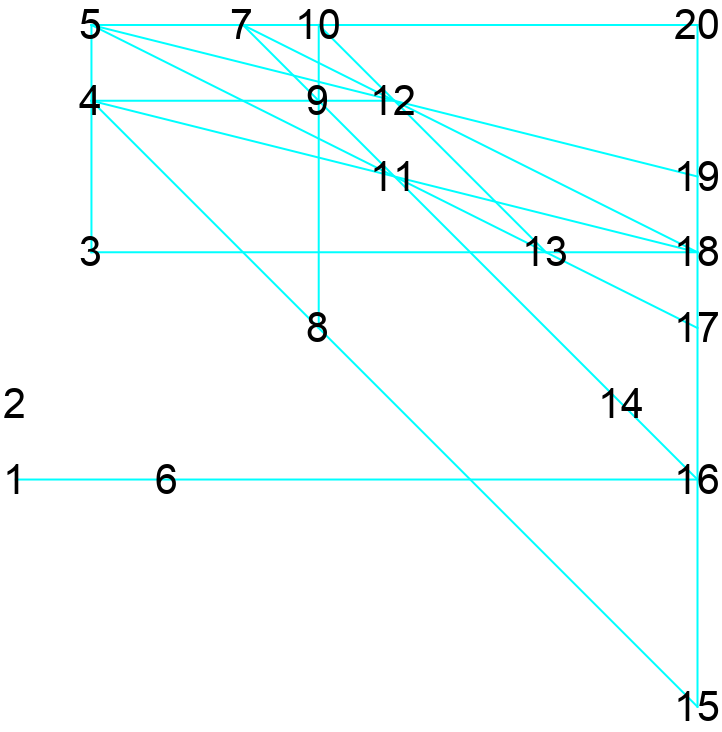## Requirements

Wolfram Language 11.3 (March 2018) or above